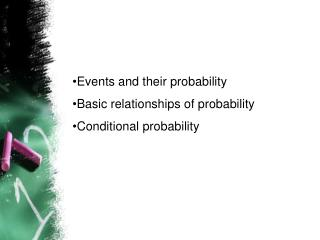DownloadDownload PresentationEvents and their probability Basic relationships of probability Conditional probability

# Events and their probability Basic relationships of probability Conditional probability

Télécharger la présentation## Events and their probability Basic relationships of probability Conditional probability

- - - - - - - - - - - - - - - - - - - - - - - - - - - E N D - - - - - - - - - - - - - - - - - - - - - - - - - - -
##### Presentation Transcript

1. Events and their probability • Basic relationships of probability • Conditional probability

2. Events and theirprobabilities • An event is a collection of sample points. • The probability of an event is equal to the sum of the probabilitiesof the sample points in the event.

3. If we can identify all the sample points in an event, and assign probabilities to each, we can compute the probability of an event.

4. Events and Their Probabilities Event M = Markey Oil is Profitable M = {(10, 8), (10, -2), (5, 8), (5, -2)} P(M) = P(10, 8) + P(10, -2) + P(5, 8) + P(5, -2) = .20 + .08 + .16 + .26 = .70

5. Events and Their Probabilities Event C = Collins Mining Profitable C = {(10, 8), (5, 8), (0, 8), (-20, 8)} P(C) = P(10, 8) + P(5, 8) + P(0, 8) + P(-20, 8) = .20 + .16 + .10 + .02 = .48

6. Basic Relationships of Probability We can use these rules to compute the probability of an even when we don’t know the probability of all the sample points. • Complement of an event • Union of two events • Intersection of 2 events • Mutually exclusive events

7. Complement of an Event The complement of event A (denoted by AC) includes all sample points outside of event A. Complement of A Event A AC Venn diagram Sample space

8. Computing Probability Using the Complement We’re trying to get you to think of probability as area or space Note that: Thus:

9. Union of Events The union of events A and B is the event containing all points in A or B or both. The union of events A ad B is denoted by Event A Event B

10. Union of Two Events Event M = Markley Oil Profitable Event C = Collins Mining Profitable MC = Markley Oil Profitable or Collins Mining Profitable MC = {(10, 8), (10, -2), (5, 8), (5, -2), (0, 8), (-20, 8)} P(MC) =P(10, 8) + P(10, -2) + P(5, 8) + P(5, -2) + P(0, 8) + P(-20, 8) = .20 + .08 + .16 + .26 + .10 + .02 = .82

11. Intersection of 2 Events • The intersection of events A and B contains all sample points in both A and B. • The intersection of events A and B is denoted by Sample space Event A Event B Intersection of events A and B

12. Intersection of 2 Events Event M = Markley Oil Profitable Event C = Collins Mining Profitable MC = Markley Oil Profitable and Collins Mining Profitable MC = {(10, 8), (5, 8)} P(MC) =P(10, 8) + P(5, 8) = .20 + .16 = .36

13. Addition Law This law provides a means of calculating the probability of event A or event B or both occur.

14. Notice we must subtract or else we will count that area twice and overstate the probability of A or B occurring Sample space Event A Event B Intersection of events A and B

15. Addition Law Event M = Markley Oil Profitable Event C = Collins Mining Profitable MC = Markley Oil Profitable or Collins Mining Profitable We know: P(M) = .70, P(C) = .48, P(MC) = .36 Thus: P(MC) = P(M) + P(C) -P(MC) = .70 + .48 - .36 = .82 (This result is the same as that obtained earlier using the definition of the probability of an event.)

16. Mutually Exclusive Events • Events A and B are said to be mutually exclusive if they have no sample points in common. • Events A and B are mutually exclusive if, when one event occurs, the other cannot occur Event A Event B Note that:

17. Conditional Probability If the probability of being promoted to detective is influenced by whether you are a man or woman, then probability is conditional

18. Conditional Probability • The notation P(A | B) reads “the probability of A given B. • Conditional probability is calculated by:

19. = Collins Mining Profitable given Markley Oil Profitable Conditional Probability Event M = Markley Oil Profitable Event C = Collins Mining Profitable We know: P(MC) = .36, P(M) = .70 Thus:

20. Multiplication Law This allows us to compute the probability of the intersection of 2 events. That is, what is the probability that both A and B will occur? Or:

21. Multiplication Law Event M = Markley Oil Profitable Event C = Collins Mining Profitable MC = Markley Oil Profitable and Collins Mining Profitable We know: P(M) = .70, P(C|M) = .5143 Thus: P(MC) = P(M)P(M|C) = (.70)(.5143) = .36 (This result is the same as that obtained earlier using the definition of the probability of an event.)

22. IndependentEvents Events A and B are said to be independent if the probability of event A is not changed by the existence of event B, and vice-versa. That is: and:

23. Multiplication Law for Independent Events The multiplication law can be used to determine if two events are independent

24. Multiplication Lawfor Independent Events Event M = Markley Oil Profitable Event C = Collins Mining Profitable Are events M and C independent? DoesP(MC) = P(M)P(C) ? We know: P(MC) = .36, P(M) = .70, P(C) = .48 But: P(M)P(C) = (.70)(.48) = .34, not .36 Hence: M and C are not independent.